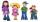# Grandfather

The grandfather is three years older than an grandmother. The average age of the grandmother, the grandfather and their seven grandchildren is 28 years. The grandchildren have a total of 105 years. Calculate how old is grandfather.

Result

o =  75

#### Solution:

o = m + 3
(m+o+7*v)/(1+1+7) = 28
v = 105/7

m-o = -3
0.111111m+0.111111o+0.777778v = 28
7v = 105

m = 72
o = 75
v = 15

Calculated by our linear equations calculator.

Leave us a comment of example and its solution (i.e. if it is still somewhat unclear...):

Showing 0 comments:Be the first to comment!#### To solve this example are needed these knowledge from mathematics:

Looking for help with calculating arithmetic mean? Looking for a statistical calculator? Do you have a linear equation or system of equations and looking for its solution? Or do you have quadratic equation?

## Next similar examples:

1. SchoolsThree schools are attended by 678 pupils. To the first attend 21 students more and to the third 108 fewer students than to second school. How many students attend the schools?
2. Boxes200 boxes have been straightened in three rows. The first was 13 more than in the second, and in the second was one fifth more than in the third one. How many boxes are in each row?
3. Ages 2A man's age is 4 times his son's age. After 5 years he will be just twice his son's age, find their ages.
4. Football seasonDalibor and Adam together scored 97 goals in the season. Adam scored 9 goals more than Dalibor. How many goals scored each?
5. Ball gameRichard, Denis and Denise together scored 932 goals. Denis scored 4 goals over Denise but Denis scored 24 goals less than Richard. Determine the number of goals for each player.
6. AntennasIf you give me two antennas will be same. If you give me again your two antenna I have a 5× so many than you. How many antennas have both mans?
7. RavensOn two trees sitting 17 ravens. If 3 ravens flew from first to second tree and 5 ravens took off from second tree then the first tree has 2 times more ravens than second tree. How many ravens was originally on every tree?
8. Two numbersWe have two numbers. Their sum is 140. One-fifth of the first number is equal to half the second number. Determine those unknown numbers.
9. Substitutionsolve equations by substitution: x+y= 11 y=5x-25
10. School yearAt the end of the school year has awarded 20% of the 250 children who attend school. Awat got 18% boys and 23% of girls. Determine how many boys and how many girls attend school.
11. Vijuviju has 40 chickens and rabbits. If in all there are 90 legs. How many rabbits are there with viju??
12. Linsys2Solve two equations with two unknowns: 400x+120y=147.2 350x+200y=144
13. EquationsSolve following system of equations: 6(x+7)+4(y-5)=12 2(x+y)-3(-2x+4y)=-44
14. MushroomsEva and Jane collected 114 mushrooms together. Eve found twice as much as Jane. How many mushrooms found each of them?
15. Two equationsSolve equations (use adding and subtracting of linear equations): -4x+11y=5 6x-11y=-5
16. Linear systemSolve a set of two equations of two unknowns: 1.5x+1.2y=0.6 0.8x-0.2y=2
17. Meanif the mean of the set of data 5, 17, 19, 14, 15, 17, 7, 11, 16, 19, 5, 5, 10, 8, 13, 14, 4, 2, 17, 11, x is -91.74, what is the value of x?